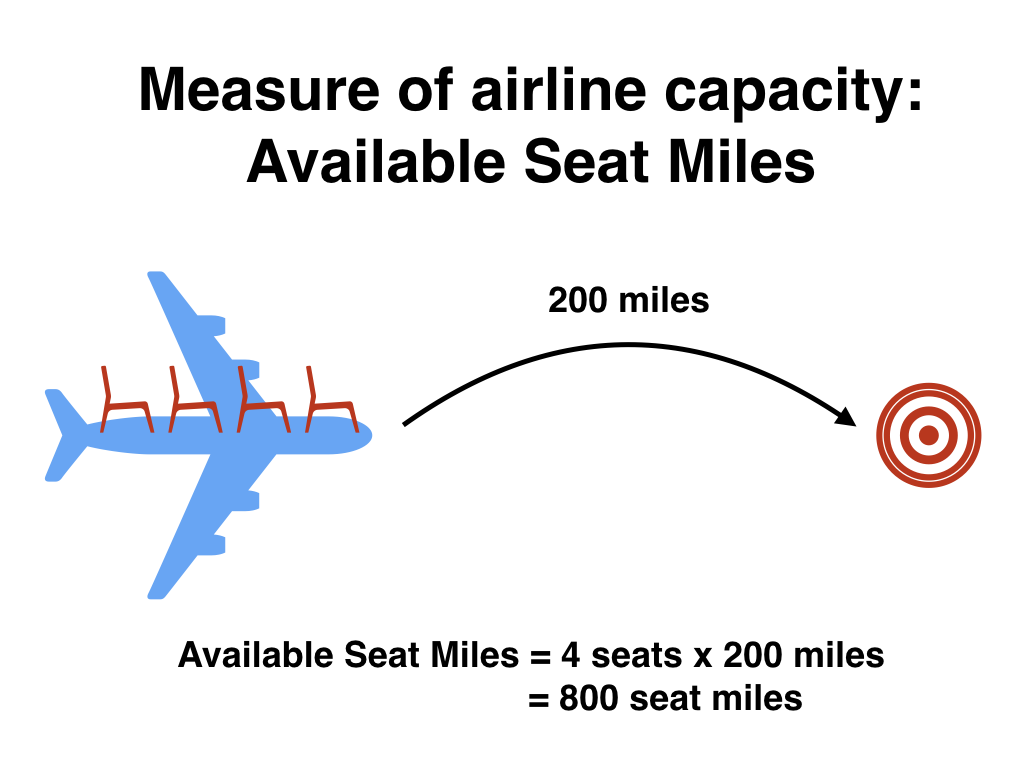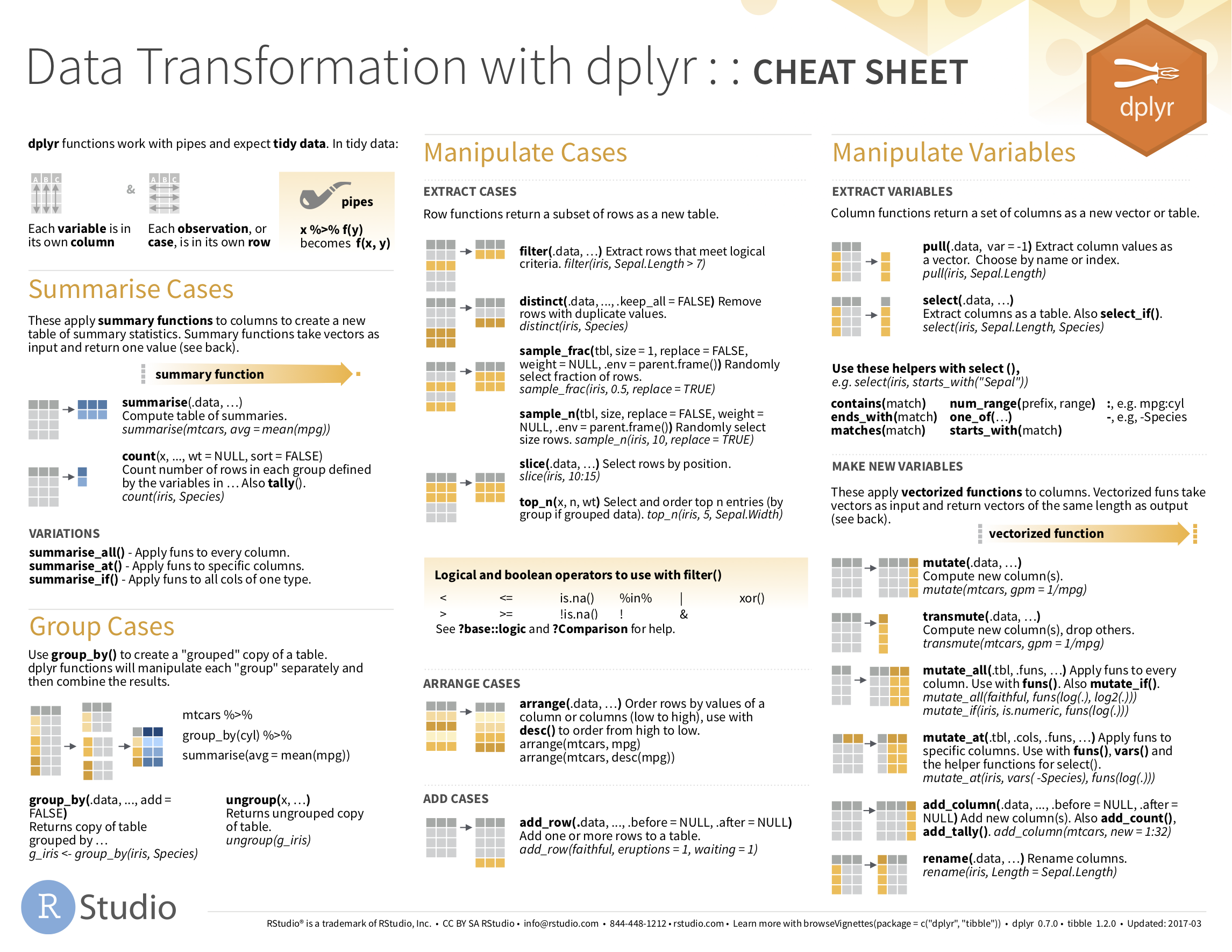## 3.9 Conclusion

### 3.9.1 Summary table

Let’s recap our data wrangling verbs in Table 3.2. Using these verbs and the pipe %>% operator from Section 3.1, you’ll be able to write easily legible code to perform almost all the data wrangling and data transformation necessary for the rest of this book.

TABLE 3.2: Summary of data wrangling verbs
Verb Data wrangling operation
filter() Pick out a subset of rows
summarize() Summarize many values to one using a summary statistic function like mean(), median(), etc.
group_by() Add grouping structure to rows in data frame. Note this does not change values in data frame, rather only the meta-data
mutate() Create new variables by mutating existing ones
arrange() Arrange rows of a data variable in ascending (default) or descending order
inner_join() Join/merge two data frames, matching rows by a key variable

Learning check

(LC3.20) Let’s now put your newly acquired data wrangling skills to the test!

An airline industry measure of a passenger airline’s capacity is the available seat miles, which is equal to the number of seats available multiplied by the number of miles or kilometers flown summed over all flights.

For example, let’s consider the scenario in Figure 3.10. Since the airplane has 4 seats and it travels 200 miles, the available seat miles are $$4 \times 200 = 800$$.FIGURE 3.10: Example of available seat miles for one flight.

Extending this idea, let’s say an airline had 2 flights using a plane with 10 seats that flew 500 miles and 3 flights using a plane with 20 seats that flew 1000 miles, the available seat miles would be $$2 \times 10 \times 500 + 3 \times 20 \times 1000 = 70,000$$ seat miles.

Using the datasets included in the nycflights13 package, compute the available seat miles for each airline sorted in descending order. After completing all the necessary data wrangling steps, the resulting data frame should have 16 rows (one for each airline) and 2 columns (airline name and available seat miles). Here are some hints:

1. Crucial: Unless you are very confident in what you are doing, it is worthwhile not starting to code right away. Rather, first sketch out on paper all the necessary data wrangling steps not using exact code, but rather high-level pseudocode that is informal yet detailed enough to articulate what you are doing. This way you won’t confuse what you are trying to do (the algorithm) with how you are going to do it (writing dplyr code).
2. Take a close look at all the datasets using the View() function: flights, weather, planes, airports, and airlines to identify which variables are necessary to compute available seat miles.
3. Figure 3.7 showing how the various datasets can be joined will also be useful.
4. Consider the data wrangling verbs in Table 3.2 as your toolbox!

An R script file of all R code used in this chapter is available here.

If you want to further unlock the power of the dplyr package for data wrangling, we suggest that you check out RStudio’s “Data Transformation with dplyr” cheatsheet. This cheatsheet summarizes much more than what we’ve discussed in this chapter, in particular more intermediate level and advanced data wrangling functions, while providing quick and easy-to-read visual descriptions. In fact, many of the diagrams illustrating data wrangling operations in this chapter, such as Figure 3.1 on filter(), originate from this cheatsheet.

In the current version of RStudio in late 2019, you can access this cheatsheet by going to the RStudio Menu Bar -> Help -> Cheatsheets -> “Data Transformation with dplyr.” You can see a preview in the figure below.FIGURE 3.11: Data Transformation with dplyr cheatsheet.

On top of the data wrangling verbs and examples we presented in this section, if you’d like to see more examples of using the dplyr package for data wrangling, check out Chapter 5 of R for Data Science (Grolemund and Wickham 2017).

### 3.9.3 What’s to come?

So far in this book, we’ve explored, visualized, and wrangled data saved in data frames. These data frames were saved in a spreadsheet-like format: in a rectangular shape with a certain number of rows corresponding to observations and a certain number of columns corresponding to variables describing these observations.

We’ll see in the upcoming Chapter 4 that there are actually two ways to represent data in spreadsheet-type rectangular format: (1) “wide” format and (2) “tall/narrow” format. The tall/narrow format is also known as “tidy” format in R user circles. While the distinction between “tidy” and non-“tidy” formatted data is subtle, it has immense implications for our data science work. This is because almost all the packages used in this book, including the ggplot2 package for data visualization and the dplyr package for data wrangling, all assume that all data frames are in “tidy” format.

Furthermore, up until now we’ve only explored, visualized, and wrangled data saved within R packages. But what if you want to analyze data that you have saved in a Microsoft Excel, a Google Sheets, or a “Comma-Separated Values” (CSV) file? In Section 4.1, we’ll show you how to import this data into R using the readr package.

### References

Grolemund, Garrett, and Hadley Wickham. 2017. R for Data Science. First. Sebastopol, CA: O’Reilly Media. https://r4ds.had.co.nz/.